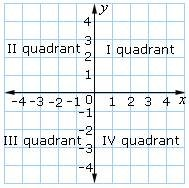The x- and y- axes divide the coordinate plane into four regions. These regions are called the quadrants.The figure below shows the four quadrants of the coordinate plane.

Ques: Identify the quadrants in which the points (- 4, 2) and (3, - 8) are located.

Choices:

A. first and second quadrants respectively
B. second and third quadrants respectively
C. second and fourth quadrants respectively